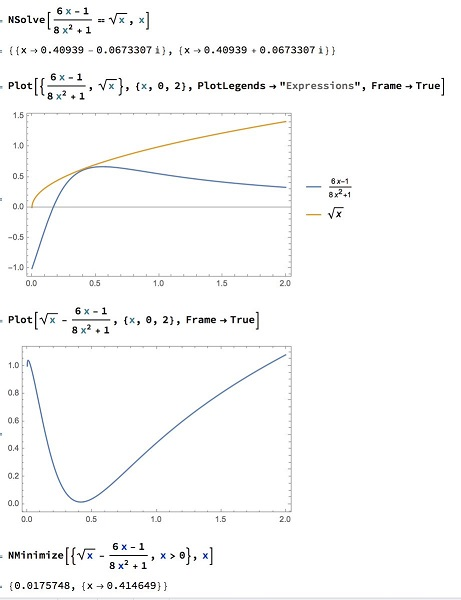# An Inequality with Two Variables from Awesome Math

### Problem### Solution

By the AM-GM inequality,

$8a^2\sqrt{a}+b^2\sqrt{a}+b^2\sqrt{b}\ge 3\left(8a^2\sqrt{a}\cdot b^2\sqrt{a}\cdot b^2\sqrt{b}\right)^{\frac{1}{3}}=6ab\sqrt{b}.$

The three terms $8a^2\sqrt{a},b^2\sqrt{a},b^2\sqrt{b}$ are never equal as would required for equality. Hence, the inequality is strict.

### Illustration### Acknowledgment

I came across this problem at the Lorian Saceanu's Easy Beautiful Math facebook group. The problem was easily traced to the awesome math site's publication Mathematical Reflections (Issue 6 2017, J421.) The problem was proposed by Adrian Andreescu and the solution is by Polyahedra, Polk State College.

The illustration is by Marcos Carreira. Amit Itagi and Nezir Hotic have independently come up with the above solution.

Inequalities in Two Variables